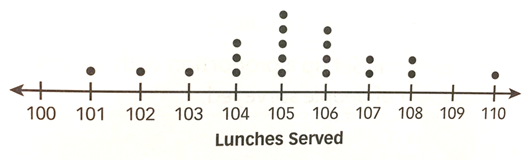Mathematics
Easy

Question

# Each dot represents 1 day. Find the total days recorded?## 92120105Hint:

## The correct answer is: 20

### It is given that each dot represents a day. The number of lunches are given on the number line.We have to find total days that are recorded.To find the total number of days, we will count the number of dots. We have to count all the dots for given number of lunch.Total number of days = ( 1 + 1 + 1 + 3 + 5 + 4 + 2 + 2 + 1)= 20Therefore, total number of days recorded  are 20.

We have to count all the dots.

### Related Questions to study#### With Turito Foundation.#### Get an Expert Advice From Turito.